Intuitionistic Logic Explorer < Previous   Next > Nearby theorems Mirrors  >  Home  >  ILE Home  >  Th. List  >  stdpc5 Unicode version

Theorem stdpc5 1521
 Description: An axiom scheme of standard predicate calculus that emulates Axiom 5 of [Mendelson] p. 69. The hypothesis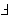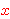can be thought of as emulating "is not free in." With this definition, the meaning of "not free" is less restrictive than the usual textbook definition; for examplewould not (for us) be free inby nfequid 1635. This theorem scheme can be proved as a metatheorem of Mendelson's axiom system, even though it is slightly stronger than his Axiom 5. (Contributed by NM, 22-Sep-1993.) (Revised by Mario Carneiro, 12-Oct-2016.) (Proof shortened by Wolf Lammen, 1-Jan-2018.)
Hypothesis
Ref Expression
stdpc5.1Assertion
Ref Expression
stdpc5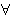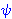Proof of Theorem stdpc5
StepHypRef Expression
1 stdpc5.1 . . 32119.21 1520 . 2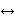32biimpi 118 1Colors of variables: wff set class Syntax hints:wi 4wal 1287wnf 1394 This theorem was proved from axioms:  ax-1 5  ax-2 6  ax-mp 7  ax-ia1 104  ax-ia2 105  ax-ia3 106  ax-5 1381  ax-gen 1383  ax-4 1445  ax-ial 1472  ax-i5r 1473 This theorem depends on definitions:  df-bi 115  df-nf 1395 This theorem is referenced by:  sbalyz  1923  ra5  2927
 Copyright terms: Public domain W3C validator